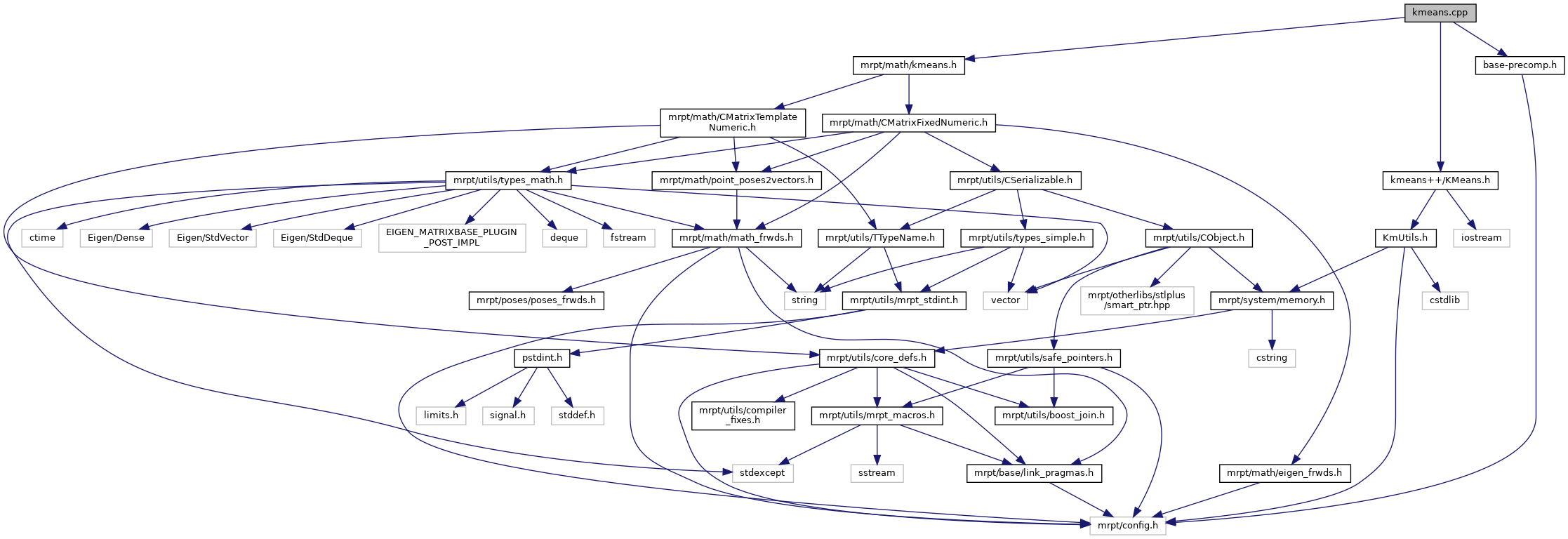Main MRPT website > C++ reference for MRPT 1.5.6
KMeans.cpp File Reference
`#include "KMeans.h"`
`#include "KmTree.h"`
`#include <mrpt/utils/mrpt_macros.h>`
`#include <sstream>`
`#include <time.h>`
`#include <vector>`
Include dependency graph for KMeans.cpp:Go to the source code of this file.

Macros

#define LOG(verbose, text)

Functions

void AddKMeansLogging (std::ostream *out, bool verbose)

void ClearKMeansLogging ()

static double GetSeconds ()

static void RunKMeansOnce (const KmTree &tree, int n, int k, int d, Scalar *points, Scalar *centers, Scalar *min_cost, Scalar *max_cost, Scalar *total_cost, double start_time, double *min_time, double *max_time, double *total_time, Scalar *best_centers, int *best_assignment)

void LogMetaStats (Scalar min_cost, Scalar max_cost, Scalar total_cost, double min_time, double max_time, double total_time, int num_attempts)

Scalar RunKMeans (int n, int k, int d, Scalar *points, int attempts, Scalar *ret_centers, int *ret_assignment)

Scalar RunKMeansPlusPlus (int n, int k, int d, Scalar *points, int attempts, Scalar *ret_centers, int *ret_assignment)

Variables

static vector< ostream * > gLogOutputs

static vector< ostream * > gVerboseLogOutputs

Macro Definition Documentation

 #define LOG ( verbose, text )
Value:
{ \
vector<ostream*> &outputs = (verbose? gVerboseLogOutputs : gLogOutputs); \
if (outputs.size() > 0) { \
ostringstream string_stream; \
string_stream << text; \
for (int i = 0; i < (int)outputs.size(); i++) \
*(outputs[i]) << string_stream.str(); \
} \
}
static vector< ostream * > gVerboseLogOutputs
Definition: KMeans.cpp:24
static vector< ostream * > gLogOutputs
Definition: KMeans.cpp:23
typedef int(WINAPI *PFNWGLRELEASEPBUFFERDCARBPROC)(HPBUFFERARB hPbuffer

Definition at line 25 of file KMeans.cpp.

Referenced by LogMetaStats(), RunKMeans(), RunKMeansOnce(), and RunKMeansPlusPlus().

Function Documentation

 void AddKMeansLogging ( std::ostream * out, bool verbose )

Definition at line 34 of file KMeans.cpp.

References gLogOutputs, and gVerboseLogOutputs.

 void ClearKMeansLogging ( )

Definition at line 39 of file KMeans.cpp.

References gLogOutputs, and gVerboseLogOutputs.

 static double GetSeconds ( )
static

Definition at line 45 of file KMeans.cpp.

Referenced by RunKMeans(), RunKMeansOnce(), and RunKMeansPlusPlus().

 void LogMetaStats ( Scalar min_cost, Scalar max_cost, Scalar total_cost, double min_time, double max_time, double total_time, int num_attempts )

Definition at line 93 of file KMeans.cpp.

References LOG.

Referenced by RunKMeans(), and RunKMeansPlusPlus().

 Scalar RunKMeans ( int n, int k, int d, Scalar * points, int attempts, Scalar * ret_centers, int * ret_assignment )

Definition at line 103 of file KMeans.cpp.

 static void RunKMeansOnce ( const KmTree & tree, int n, int k, int d, Scalar * points, Scalar * centers, Scalar * min_cost, Scalar * max_cost, Scalar * total_cost, double start_time, double * min_time, double * max_time, double * total_time, Scalar * best_centers, int * best_assignment )
static

Definition at line 53 of file KMeans.cpp.

Referenced by RunKMeans(), and RunKMeansPlusPlus().

 Scalar RunKMeansPlusPlus ( int n, int k, int d, Scalar * points, int attempts, Scalar * ret_centers, int * ret_assignment )

Definition at line 152 of file KMeans.cpp.

Variable Documentation

 vector gLogOutputs
static

Definition at line 23 of file KMeans.cpp.

 Page generated by Doxygen 1.8.6 for MRPT 1.5.6 Git: 4c65e84 Tue Apr 24 08:18:17 2018 +0200 at mar abr 24 08:26:17 CEST 2018